# COS 426 Assignment #3:Ray Tracing (Part 1)

## Basic Ray Generation

### Generate a ray for each pixel

Create an R3Ray through points on a regular grid of pixels on the view plane, as defined by the camera parameters (eye point, towards direction, up direction, xfov, and yfov).
NOTE: xfov and yfov are the half-angles along the "left" direction and "up" direction, respectively.For this discussion, assume the pixel through which the ray is constructed is at coordinates (i,j)
To determine the ray boundary along the "x" direction (left/right), add and subtract (right*tan(xfov) + towards) to the camera's position
P1 = P0 + towards - tan(xfov)*right (left boundary)
P2 = P0 + towards + tan(xfov)*right (right boundary)

FinalPoint = P1 + ((i + 0.5)/width) * (P2 - P1) (interpolate between the left and right boundary to figure out the direction of the ray through the current pixel at (i,j) on the viewing plane)

Now the camera needs to be moved up along the "up" direction based on j. Do a similar process by adding to final point:
FinalPoint = FinalPoint + up * (j - height/2) / (height/2) * tan(yfov);
Repeat this process for all pixels on the grid

I made a debugging routine in rayview so that if the user presses "R," then these rays are drawn from the camera position and saved (a 10x10 pixel grid is assumed so that only 100 example rays have to be calculated and re-rendered each time). Then user can then move the camera around and view the rays cast from that position from a different angle. I thought this was easier than manipulating two different cameras at once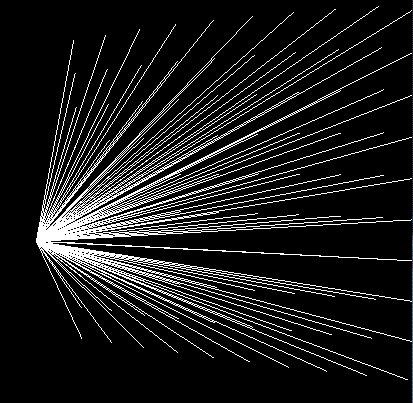## Ray-Primitive Intersections

In these examples, I cast the rays and find the intersections. If an intersection occurs, I draw the pixel as white. Otherwise, the pixel is black. This is a preliminary step to more complicated lighting schemes later.

### Intersect a ray with a sphere

`void R3Intersects::rayIntersectSphere(R3Ray* ray, R3Sphere* sphere)`
Figure out where the ray intersects the surface of the sphere (if it does), and the position, normal, and parametric value (t) at the first intersection point.
Here's an algorithm I pulled from one of the lecture slides: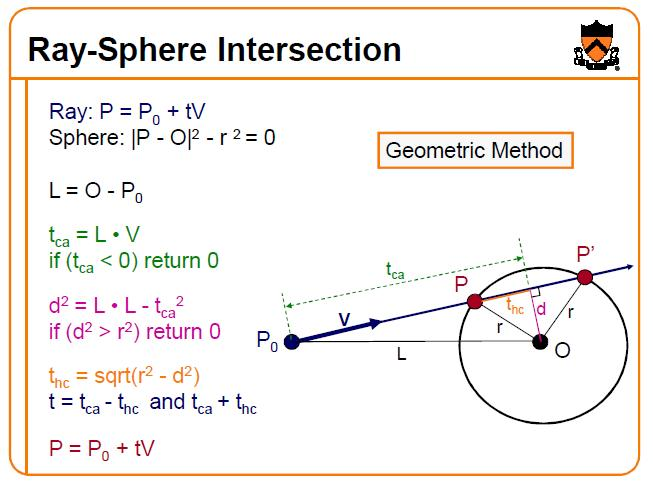Picture courtesy of Tom Funkhouser

• tca is the distance to the center projected onto V. If this is negative, it means that the ray is facing away from the sphere and, therefore, does not intersect
• d^2 is the perpendicular distance from the ray to the center of the sphere
• thc is the other side of the right triangle that's formed between r and d, and it's used to help determine the two possible t's of intersection along the sphere. Take the closest in this implementation intersection (tca - thc).
• The normal is along the direction P - O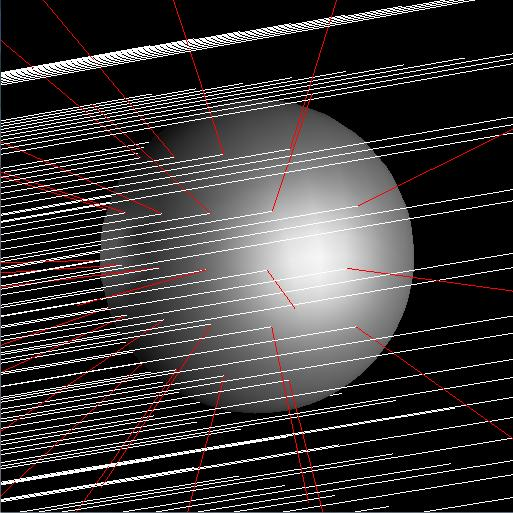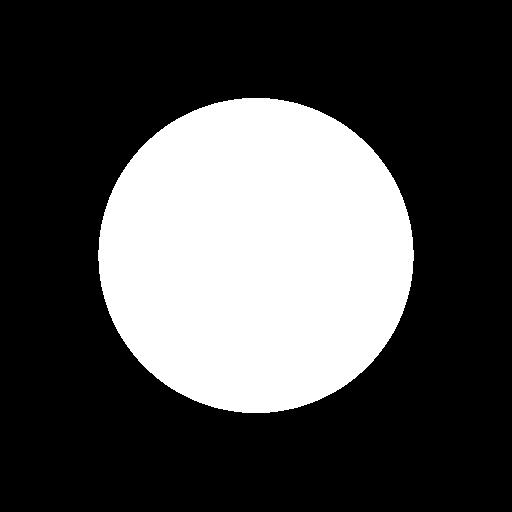NOTE: My rayview draws the normals in red

### Intersect a ray with an axis-aligned box

`void R3Intersects::rayIntersectBox(R3Ray* ray, R3Box* box)`
Intersect a ray with a box. The normals are also axis-aligned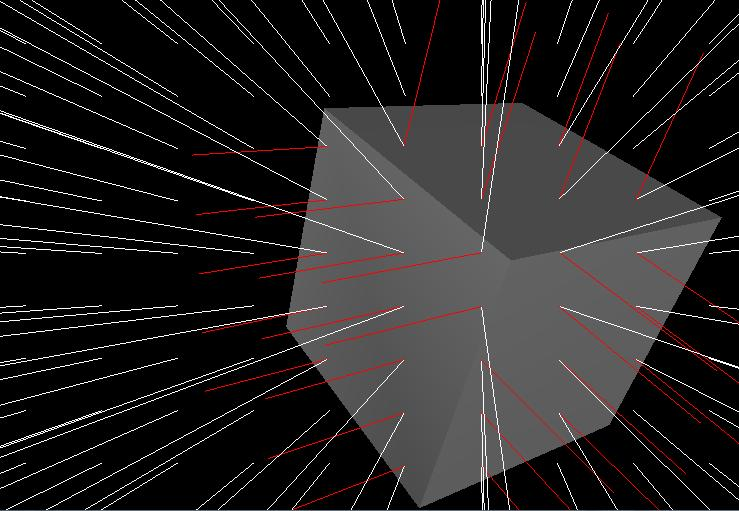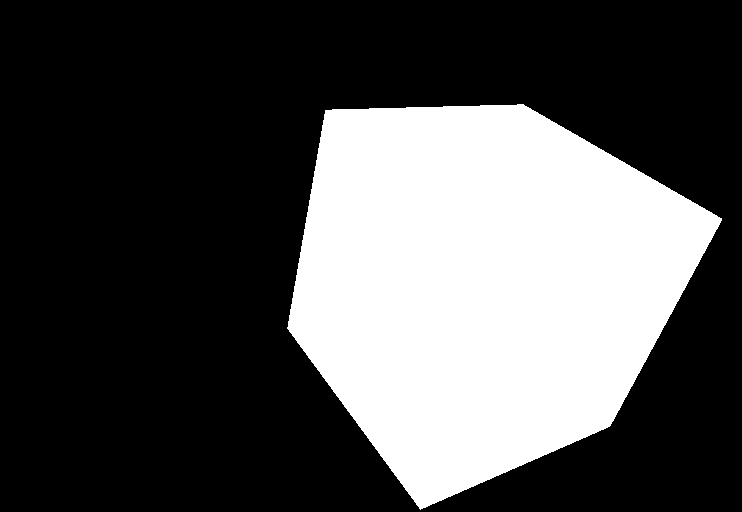### Intersect a ray with a triangle mesh

`void R3Intersects::rayIntersectTriMesh(R3Ray* ray, R3Mesh* mesh)`
Loop through all of the triangles in the triangle mesh, and check, one-by-one, to see if the ray intersects it. For each face, first, do a ray-plane intersection. If the intersection occurs, check to see if it is within the triangle's boundaries. Do this by creating a triangular prism between the ray's endpoint and the triangle, and determining if the ray-plane intersection point is within that prism. If an intersection does occur, then the normal of the intersection is simply the normal of that face, which has already beeen calculated (assuming that the face's vertices have been specified in counter-clockwise order).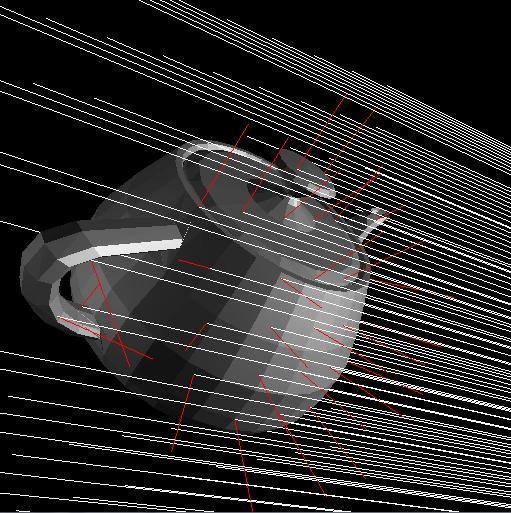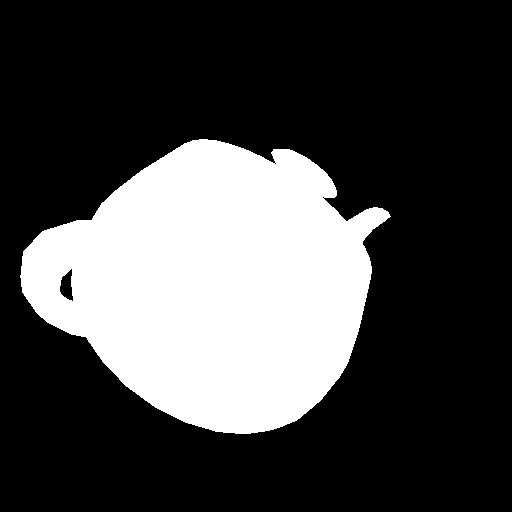### Intersect a ray with an axis-aligned cylinder

`void R3Intersects::rayIntersectCylinder(R3Ray* ray, R3Cylinder* cylinder)`
Determine the intersection between a ray and an axis-aligned cylinder that has a height h, a radius r, and a center c. Note that the axis of the cylinder is assumed to be the y-axis.
If the endpoint of the ray is above the top of the cylinder, then it's possible for an intersection with the top circle of the sphere to occur, so check this first if this is the case. Likewise, if the endpoint of the ray is below the bottom of the cylinder, then it's possible for an intersection with the bottom circle of the sphere to occur, so check this. If these intersections do occur, then their normals are (0, 1, 0) and (0, -1, 0), respectively.

If neither one of the circle intersections occurs, then proceed to calculate the intersection along the rest of the cylinder. Treat it as if it were an infinite cylinder first, and then check to see if the calculated intersection is within the boundaries determined by the height.

To do this, follow the same exact algorithm as in the ray-sphere case, except do it in 2D along the XZ plane only, so it turns into a ray-circle intersection. That is, project the ray onto the XZ plane and do an intersection between that projected ray and a circle centered at the cylinder's center and with the cylinder's radius. If this intersection occurs, then the normal will be the intersection point minus the center of the cylinder, projected onto the XZ plane. Also, when calculating what t is (to help determine the point of intersection), divide the t between the projected ray and circle by the cosine of the angle between the original ray and the XZ plane to get the correct value (effectively un-doing the projection).

If there is an intersection point, check to make sure it is within the height boundaries before accepting it.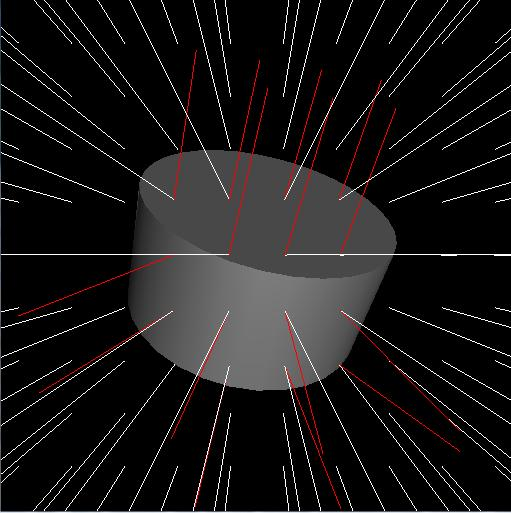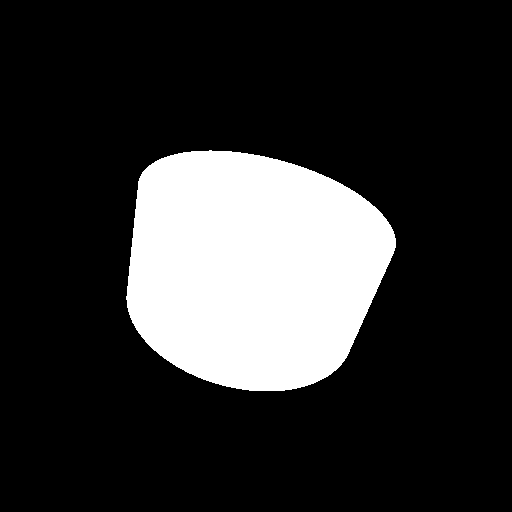### Intersect a ray with an axis-aligned cone

`void R3Intersects::rayIntersectCone(R3Ray* ray, R3Cone* cone)`
Intersect a ray with an axis-aligned (along the y-axis) cone, which has radius r, height h, and endpoint yend.First of all, if the ray has an endpoint below the bottom circle of the cone, check to see if the ray intersects it (exact process as in the cylinder case). If it does, then the process is finished and the normal is (0, -1, 0). Otherwise, proceed to calculate the intersection along an infinite cone, and then make sure the intersection is within the height boundaries:

To satisfy the locus of points along an infinite, double-ended cone, the point has to be along a circle parallel to the XZ plane with varying radius based on height. The radius given based on height y is (r / h) * (yend - y). Let k = r/h, and let this new radius be rnew. So to satisfy the cone,
x2 + z2 = rnew2
x2 + z2 = k2*(yend - y)2

Now plug in the ray (X0 + t*Vx, Y0 + t*Vy, Z0 + t*Vz) and solve for t:

(X0 + tVx)2 + (Z0 + tVz)2 = k2*(y0 + tVy - yend)2
(X0 + tVx)2 + (Z0 + tVz)2 = k2*((y0 - yend) + tVy)2
Let yk = y0 - yend. Then, expanding and collecting terms, the quadratic equation turns into this:
t2(Vx*Vx + Vz*Vz - k*k*Vy*Vy) + 2t(Vx*X0 + Vz*Z0 - k*k*yk*Vy) + (X0*X0 + Z0*Z0 - k*k*yk*yk) = 0
So now it's just a matter of applying the quadratic formula and solving for t. If the discriminant is negative or t turns out to be negative, then an intersection did not occur.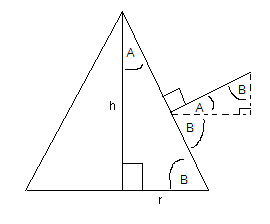To calculate the normal, first subtract the cone's center from the ray's endpoint, and project it along the XZ plane. To determine the y-component of the normal, multiply the magnitude of the xz part of the normal by tan(A) (as shown in the diagram above), where tan(A) is (r/h).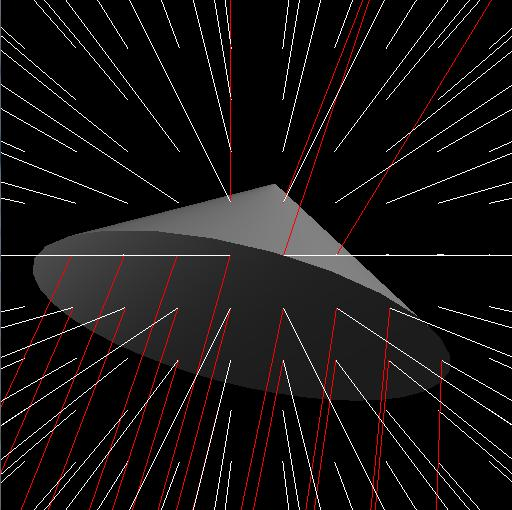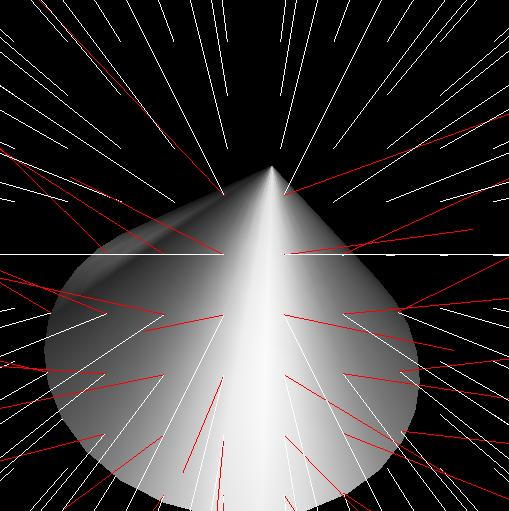## Ray-Scene Intersections

### Intersect a ray with a scene graph

```void R3Intersects::rayIntersectScene(R3Ray* ray, R3Scene* scene)
void R3Intersects::rayIntersectNode(R3Ray* ray, R3Node* node, R3Scene* scene, R3Matrix transforms)```
Implement a version of R3Intersets that takes in a ray and a scene as arguments and returns whether or not the ray intersects the scene, and if so what is the scene graph node, position, normal, and parametric value t at the first intersection point. This function should traverse the scene graph hierarchy recursively, intersecting the ray with all nodes, and returning the intersection with minimal parametric value (t) along the ray.

I used rayIntersectScene to start the recursive calls to rayIntersectNode. At each node, more calls are made to rayIntersectNode for every child of the node. The transformations between each node in the scene graph are multiplied together as the recursion goes along. The function checks to make sure all of the t values are between specified min and max view distances by projecting every ray onto the center direction of the view frustrum.

This is how I was able to get all of the black/white pictures above, so no screenshot is needed for now

### Handle scene traversals with modeling transformations

I keep a matrix that's the product of all of the transformations that have been done so far going along the scene graph. Every time the recursion goes a step deeper, multiply this matrix on the right by the newest transformation. Before checking the ray-primitive intersection at that step, multiply the ray by the inverse of the accumulation transformation matrix. Then, after the intersection has been performed, multiply the calculated intersection point and the normal by the accumulation matrix to put them back in the regular world coordinates, and recalculate t (since scaling may have occurred).

Here's an example below, using hierarchy.scn, which uses such transformations to describe different objects (mostly just translations in this case).## Expected Point Earnings For Part 1

Bold means required
 Operation Points Generate a ray for each pixel 1 Intersect a ray with a sphere 1 Intersect a ray with an axis-aligned box 1 Intersect a ray with a triangle mesh 2 Intersect a ray with an axis-aligned cylinder 2 Intersect a ray with an axis-aligned cone 2 Intersect a ray with a scene 1 Handle scene traversals with modeling transformations 1 Total 11